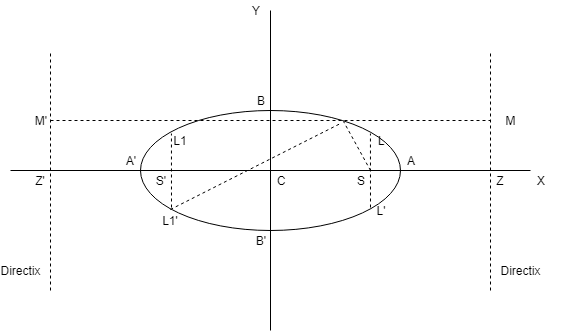# Equation of Ellipse in Standard Form

## Equation of Ellipse in Standard Form

The equation of ellipse in standard form referred to its principal axes along the coordinate axes is

$$x^2\over a^2$$ + $$y^2\over b^2$$ = 1,

where a > b & $$b^2$$ = $$a^2(1 – e^2)$$ $$\implies$$ $$a^2$$ – $$b^2$$ = $$a^2e^2$$.

where e = eccentricity (0 < e < 1)

Foci : S = (ae, 0) & S’ = (-ae, 0)

Vertices : A’ = (-a, 0) and  A’ = (a, 0)#### (a) Equation of directrix of Ellipse :

x = $$a\over e$$  and  x = $$-a\over e$$

#### (b) Major axis of Ellipse :

The line segment A’A in which the foci S’ & S lie is of length 2a & is called the major axis (a > b) of the ellipse. The Point of intersection of major axis with directrix is called the foot of the directrix(z).

#### (c) Minor axis of Ellipse :

The y-axis intersects the ellipse in the points B’ = (0,-b) & B = (0,b). The line segment B’B of length 2b (b < a) is called the minor axis of the ellipse.

Both the axes minor and major together are called Principal Axes of the ellipse.

#### (d)  Double ordinate of Ellipse :

A chord perpendicular to major axis is called double ordinate of ellipse.

#### (e)  Latus Rectum of Ellipse :

The focal chord perpendicular to major axis is called the latus rectum of ellipse.

(i)  Length of latus rectum(LL’) = $$2b^2\over a$$ = $${(minor axis)}^2\over {major axis}$$ = 2a(1 – $$e^2$$)

(ii) Equation of latus rectum : x = $$\pm$$ae

(iii)  Ends of latus rectum are L(ae, $$b^2\over a$$), L'(ae, -$$b^2\over a$$), L1(-ae, $$b^2\over a$$),
L1′(-ae, -$$b^2\over a$$)

#### (f)  Eccentricity of Ellipse :

e = $$\sqrt{1 – {b^2\over a^2}}$$

Example : Find the equation of ellipse in standard form having center at (1, 2), one focus at (6, 2) and passing through the point (4, 6).

Solution : With center at (1, 2), the equation of the ellipse is $$(x – 1)^2\over a^2$$ + $$(y – 2)^2\over b^2$$ = 1. It passes through the point (4, 6)

$$\implies$$ $$9\over a^2$$ + $$16\over b^2$$ = 1 …..(i)

Distance between focus and center = (6 – 1) = 5 = ae

$$\implies$$ $$b^2$$ = $$a^2$$ – $$a^2e^2$$ = $$a^2$$ – 25 …..(ii)

Solving (i) and (ii)

we get $$a^2$$ = 45 and $$b^2$$ = 20

Hence, the equation of the ellipse is $$(x – 1)^2\over 45$$ + $$(y – 2)^2\over 20$$ = 1

### Related Questions

Find the equation of ellipse whose foci are (2, 3), (-2, 3) and whose semi major axis is of length $$\sqrt{5}$$.

What is the parametric equation of ellipse ?

The foci of an ellipse are $$(\pm 2, 0)$$ and its eccentricity is 1/2, find its equation.